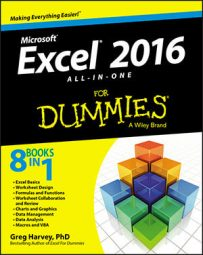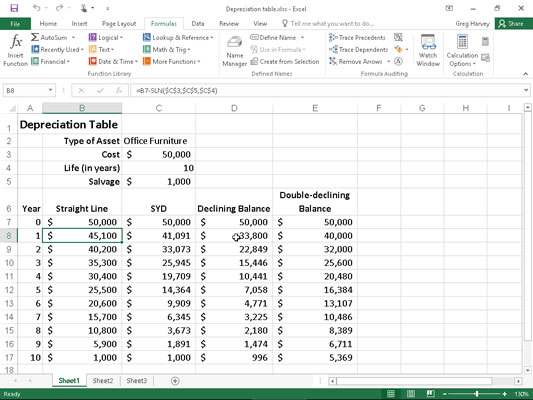##### Excel 2016 All-in-One For DummiesExcel 2016 lets you choose from four different depreciation functions, each of which uses a slightly different method for depreciating an asset over time. These built-in depreciation functions found on the Financial button's drop-down menu on the Formulas tab of the Ribbon include the following:

• SLN(cost,salvage,life) to calculate straight-line depreciation

• SYD(cost,salvage,life,per) to calculate sum-of-years-digits depreciation

• DB(cost,salvage,life,period,[month]) to calculate declining balance depreciation

• DDB(cost,salvage,life,period,[factor]) to calculate double-declining balance depreciation

As you can see, with the exception of the optional month argument in the DB function and the optional factor argument in the DDB function, all the depreciation functions require the cost, salvage, and life arguments, and all but the SLN function require a period argument as well:

• Cost is the initial cost of the asset that you're depreciating.

• Salvage is the value of the asset at the end of the depreciation (also known as the salvage value of the asset).

• Life is the number of periods over which the asset is depreciating (also known as the useful life of the asset).

• Per or period is the period over which the asset is being depreciated. The units that you use in the period argument must be the same as those used in the life argument of the depreciation function so that if you express the life argument in years, you must also express the period argument in years.

Note that the DB function accepts an optional month argument. This argument is the number of months that the asset is in use in the first year. If you omit the month argument from your DB function, Excel assumes the number of months of service to be 12.

When using the DDB function to calculate the double-declining balance method of depreciation, you can add an optional factor argument. This argument is the rate at which the balance declines in the depreciation schedule. If you omit this optional factor argument, Excel assumes the rate to be 2 (thus, the name double-declining balance).

The figure contains a Depreciation table that uses all four depreciation methods to calculate the depreciation of office furniture originally costing \$50,000 to be depreciated over a 10-year period, assuming a salvage value of \$1,000 at the end of this depreciation period.A Depreciation table showing 10-year depreciation of an asset using various methods.

The Formula bar here shows the SLN formula entered into cell B8:

`=B7-SLN(\$C\$3,\$C\$5,\$C\$4)`

This formula subtracts the amount of straight-line depreciation to be taken in the first year of service from the original cost of \$50,000. (This value is brought forward from cell C3 by the formula =C3.) After creating this original formula in cell B8, the Fill handle was used to copy it down to cell B17, which contains the final salvage value of the asset in the 10th year of service.

Cell C8 contains a similar formula for calculating the sum-of-years-digits depreciation for the office furniture. This cell contains the following formula:

`=C7-SYD(\$C\$3,\$C\$5,\$C\$4,\$A8)`

This formula subtracts the amount of sum-of-years-digits depreciation to be taken at the end of the first year from the original cost of \$50,000 in cell C7 (also brought forward from cell C3 by the formula =C3). After creating this original formula in cell C8, the Fill handle was again used to copy it down to cell C17, which also contains the final salvage value of the asset in the 10th year of service.

The same basic procedure was used to create the formulas using the DB and DDB depreciation methods in the cell ranges D8:D17 and E8:E17, respectively. Cell D8 contains the following DB formula:

`=D7-DB(\$C\$3,\$C\$5,\$C\$4,\$A8)`

Cell E8 contains the following DDB formula:

`=E7-DDB(\$C\$3,\$C\$5,\$C\$4,\$A8)`

Note that, like the SYD function, both of these depreciation functions require the use of a period argument, which is supplied by the list of years in the cell range A8:A17. Note also that the value in cell C4, which supplies the life argument to the SYD, DB, and DDB functions, matches the year units used in this cell range.